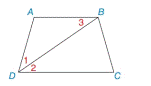Chapter 2.4, Problem 18E### Elementary Geometry for College St...

6th Edition
Daniel C. Alexander + 1 other
ISBN: 9781285195698

#### Solutions

Chapter
Section### Elementary Geometry for College St...

6th Edition
Daniel C. Alexander + 1 other
ISBN: 9781285195698
Textbook Problem
1 views

# Given: A B ¯ ∥ D C ¯ D B → bisects ∠ A D C m ∠ 1 = 36 ° Find: m ∠ ATo determine

To find:

The value of mA.

Explanation

Given:

The given statement is,

AB¯DC¯ and DB bisects ADC and m1=36°.

Figure (1)

Properties:

(1) If two parallel lines are cut by a transversal, then the pair of interior angles are congruent.

(2) In a triangle, the sum of the measures of the interior angles is 180°.

Calculation:

The given statement is,

So,

m1=m212

AB¯DC¯

2 and 3 are two interior angles.

23

Use Transitive Property of Congruence

### Still sussing out bartleby?

Check out a sample textbook solution.

See a sample solution

#### The Solution to Your Study Problems

Bartleby provides explanations to thousands of textbook problems written by our experts, many with advanced degrees!

Get Started

#### In Exercises 1-6, simplify the expression. 2. 2a23ab9b22ab2+3b3

Applied Calculus for the Managerial, Life, and Social Sciences: A Brief Approach

#### 44. Does the following matrix have an inverse?

Mathematical Applications for the Management, Life, and Social Sciences

#### Determine the infinite limit. limx(/2)+1xsecx

Single Variable Calculus: Early Transcendentals, Volume I